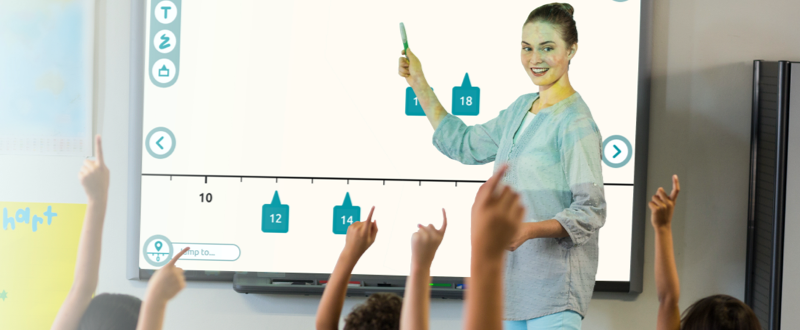## Jump into this new workshop with your children today to learn how valuable a number line can be!

Skills: Arithmetic Operations, Number Line

### Learning Objectives

Visualise numbers (integers, fractions, decimals and negative numbers) by placing them on a number line. Use a number line to represent the four arithmetic operations.

### Mathematical Background

The number line is a visual model of the real number system. It represents numbers as points on a line. Used extensively in the primary grades to visualise integers and their order, it can also be used to visualise the operations of addition and subtraction. Later in the higher grades, it serves to visualise skip-counting, in preparation for multiplication, and to visualise fractions by partitioning the unit interval into equal parts. At even higher grades, the number line also serves to model negative numbers. When using the number line as a model for the number system, the “greater than” and “less than” relations have a geometric representation as “left of” and “right of” and differences between numbers are naturally converted into distances between points, thus preparing children for more complex mathematics in the grades to come.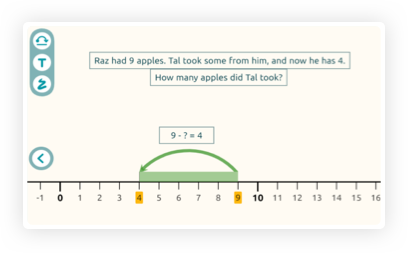### Pay Attention to

This workshop's key features include:

• Great flexibility for many grade levels uses including an introduction to whole numbers, addition, addition with unknowns, subtraction, multiplication as repeated addition, number series, and more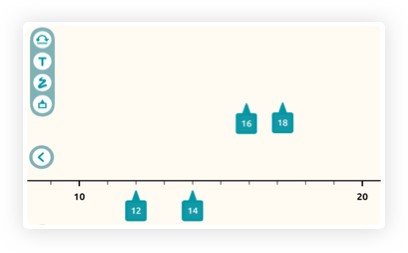• Partially transparent arrows, which allows superimposing them, for example, to demonstrate compound operations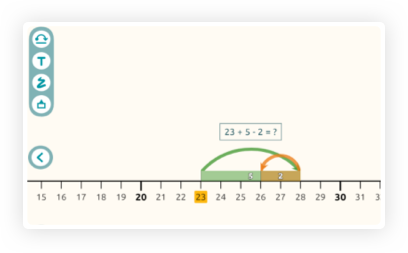• Arrows that can be duplicated, for example, to illustrate skip-counting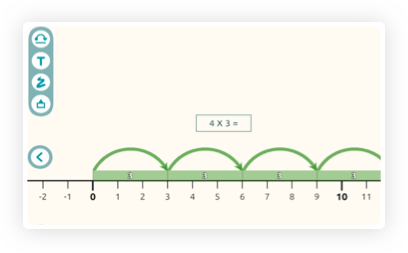• Unlimited scrolling along the number line to help children get a feel for the infinity of the number system
• Coming soon: save & load features, so that teachers can prepare their own lessons and upload them in class and premade sets with attached lesson plans